# 【c++】万字长文，浅析c++继承特性

+关注继续查看

## 1. 继承的概念和定义

### 1.2 定义

class Person
{
public:
void Print()
{
cout << "name:" << _name << endl;
cout << "age:" << _age << endl;
}
protected:
string _name = "peter"; // 姓名
int _age = 18; // 年龄
};


class Student : public Person
{
protected:
int _stuid; // 学号
};



#### 1.2.1 定义格式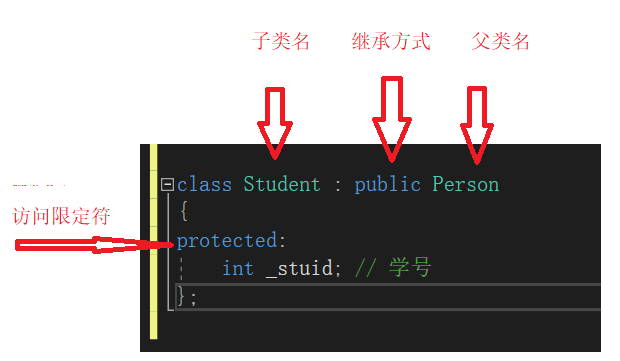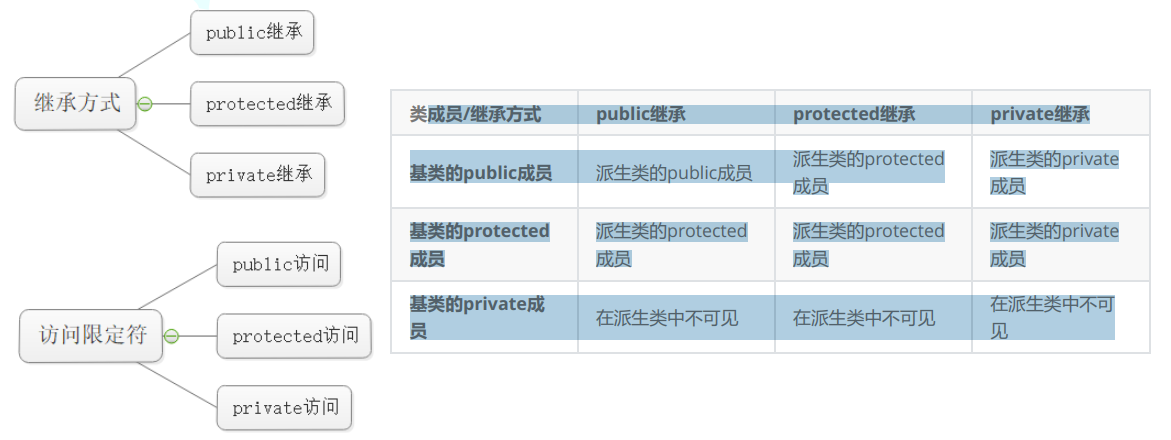## 2.基类和派生类对象赋值转换（##）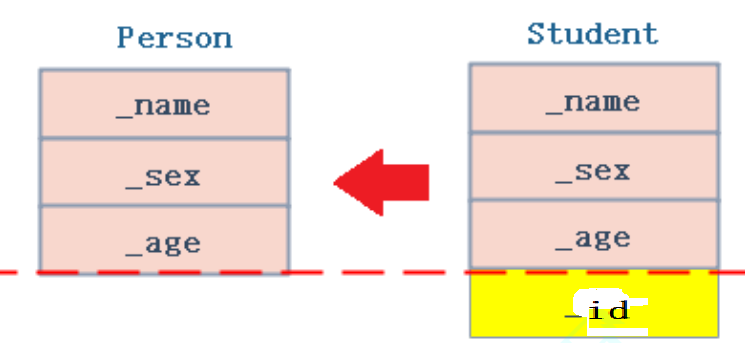1. 基类对象不能赋值给派生类对象
// 子类对象可以赋值给父类对象/指针/引用
Student s;
Person p1;
Person p2 = s;
Person* p3 = &s;
Person& p4= s;

//父类对象不能给子类对象赋值
s=p1;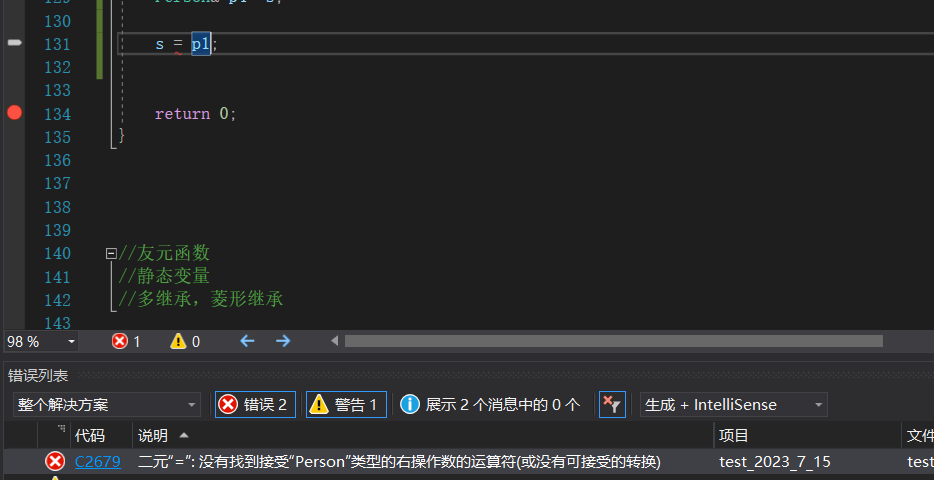## 3. 继承中的变量和函数隐藏(#)

1. 在继承体系中基类和派生类都有独立的作用域。
2. 子类和父类中有同名成员，子类成员将屏蔽父类对同名成员的直接访问，这种情况叫隐藏，
也叫重定义。（在子类成员函数中，可以使用 基类::基类成员 显示访问）
3. 需要注意的是如果是成员函数的隐藏，只需要函数名相同就构成隐藏。
4. 注意在实际中在继承体系里面最好不要定义同名的成员。
class A {
public:
int val = 10;

void fun()
{
cout << "A::fun()" << endl;
}
};

class B : public A
{
public:
int val = 20;
void fun()
{
cout << "B::fun()" << endl;
}
};

int main()
{
A a;
B b;
cout<<a.val<< " "<<b.val<<endl;    //    10     20
a.fun();    //打印出  "A::fun()"
b.fun();    //打印出  "B::fun()"
//作为子类 ，也可以显示调用父类的数据

b.A::a;   //打印 10
b.A::fun();   //   "A::fun()"

return 0;
}


fun()函数构成的不是函数重载，因为他们不在同一个作用域中，父类作用域和子类是不同的作用域，他们构成了重定义。

## 4.派生类的默认成员函数（###）

1. 派生类的构造函数必须调用基类的构造函数初始化基类的那一部分成员。如果基类没有默认
的构造函数，则必须在派生类构造函数的初始化列表阶段显示调用。
1. 派生类的拷贝构造函数必须调用基类的拷贝构造完成基类的拷贝初始化。
2. 派生类的operator=必须要调用基类的operator=完成基类的复制

class  Person {
public:
Person() {
cout << "Person()" << endl;
}
Person(const string& n, int a)
:name(n)
, age(a)
{}
Person(const Person& p)
{
cout << "Person(const Person& p)" << endl;
}
Person& operator=(const Person& p) {
cout << "operator=(const Person& p)" << endl;
return *this;
}
~Person()
{
cout << "~Person()" << endl;
}

string name="张三";
int age=18;
};

class  Student : public  Person
{
public:
Student(int id = 123456)
:id(123456)
{}
int id;
};


Student s;
Student s1 = s;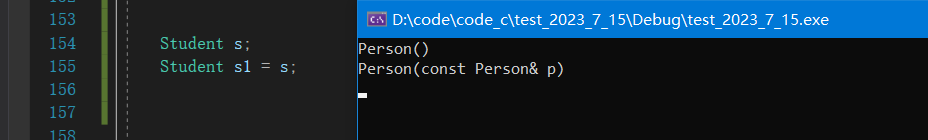Student s1;
Student s2;
s1 = s2;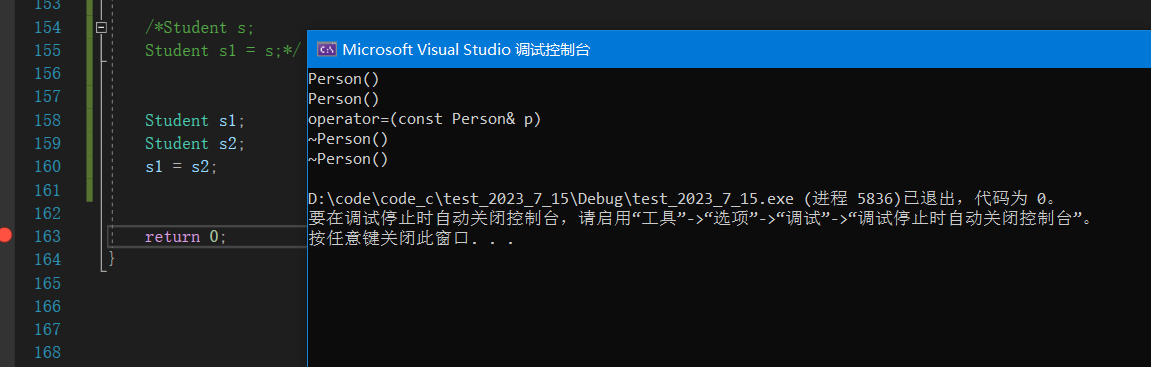class  Student : public  Person
{
public:
Student(int id = 123456)
:id(123456)
{}
Student(const string& s, int a, int num)
:Person(s, a)
, id(num)
{}
Student(const Student& s)
:Person(s)//切片后在调用父类的拷贝构造
,id(s.id)
{}
Student operator=(const Student& s)
{
Person::operator=(s);
id = s.id;
}

~Student()
{
Person::~Person();

);
}
int id;
};


~Student()
{
~Person();
_id=0;
}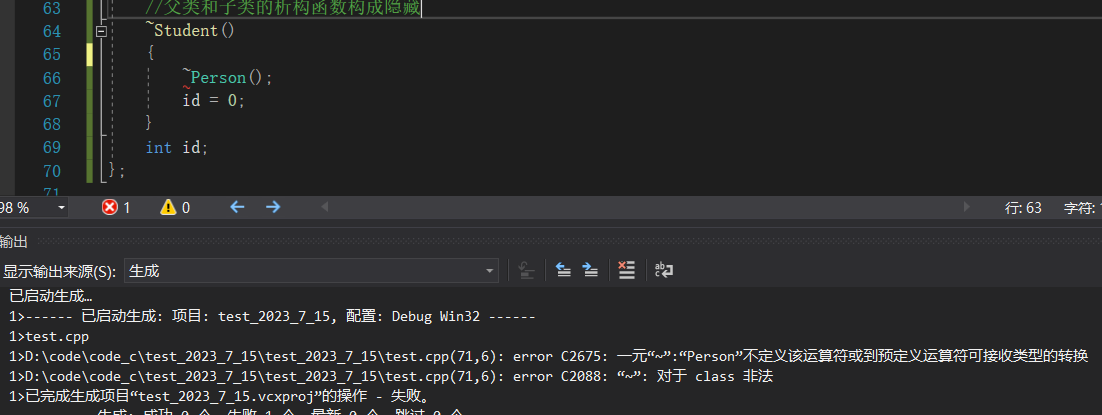~Student()
{
Person::~Person();
_id=0;
}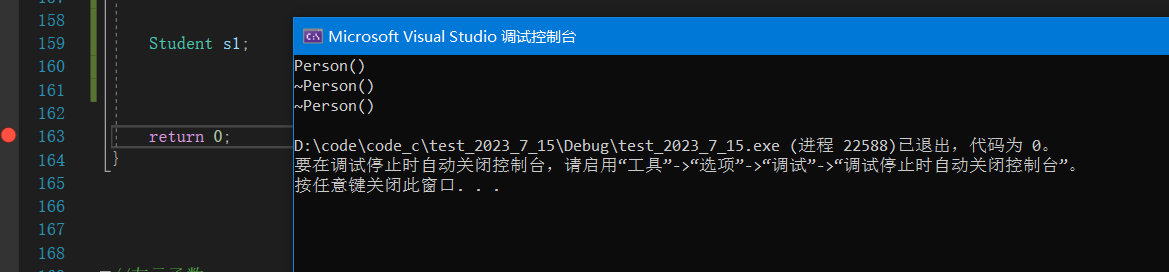1. 子类的析构会自动调用父类的析构函数，如果我们自己显示调用，会造成父类资源多次释放，在涉及动态资源开辟时会报错。
2. 无需我们显示调用父类的析构函数，因为编译器要保证析构的顺序。
1. 析构时是先析构子类区别于父类的资源，在调用父类的析构函数析构父类。
2. 构造时先父类再子类，析构时先子类再父类，符合栈的规则(都是定义在栈区上的变量)

## 5.友元函数和静态成员

### 5.2.静态成员

class  Person {
public:
static int count;
public:
Person() {
cout << "Person()" << endl;
count++;
}
Person(const string& n, int a)
:name(n)
, age(a)
{
count++;
}
Person(const Person& p)
{
cout << "Person(const Person& p)" << endl;
count++;
}
}

int Person::count = 0;// 类外对静态变量进行初始化


Person p1;
Person P2;
Person s3=s2;
Student s1;
Student s2;
Student s3=s2;

cout<<Person::count<<endl;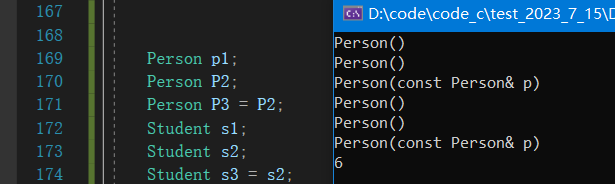## 6.菱形继承（###）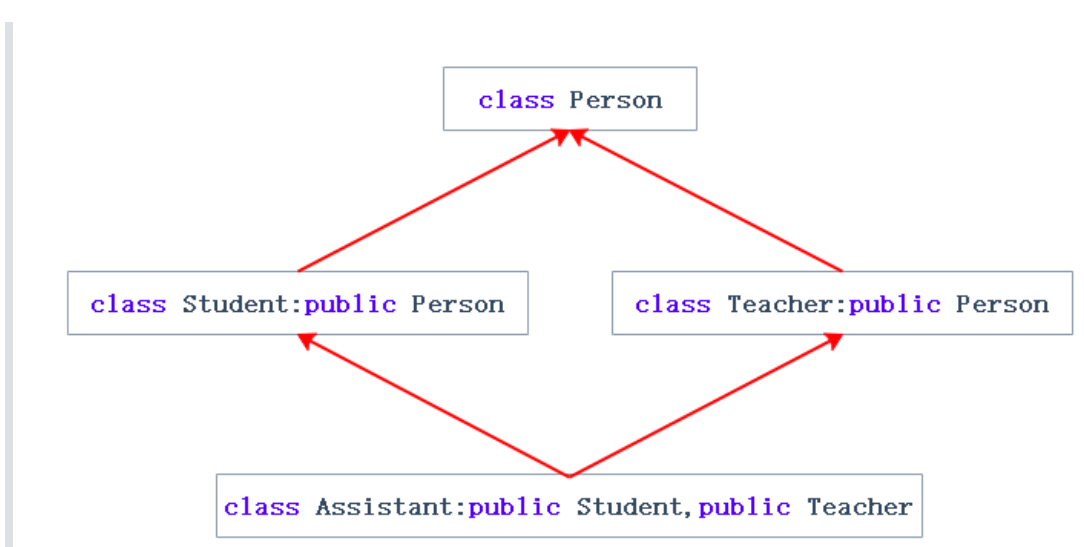class Person{....};

class Student :public Person
{....};
class Teacher :public Person
{....};

class Assistant :public Student public Teacher
{....};


Student 类继承了Person 类中的 _name=“小张” ，_age =18;

Teacher 类继承了Person 类中的 _name=“老张”，_age=18;

class Person
{
public:
string _name; // 姓名
};
class Student : public Person
{
protected:
int _num; //学号
};
class Teacher : public Person
{
protected:
int _id; // 职工编号
};
class Assistant : public Student, public Teacher
{
protected:
string _majorCourse; // 主修课程
};

int main()
{
Assistant a;
a._name = "张三";
return 0;
}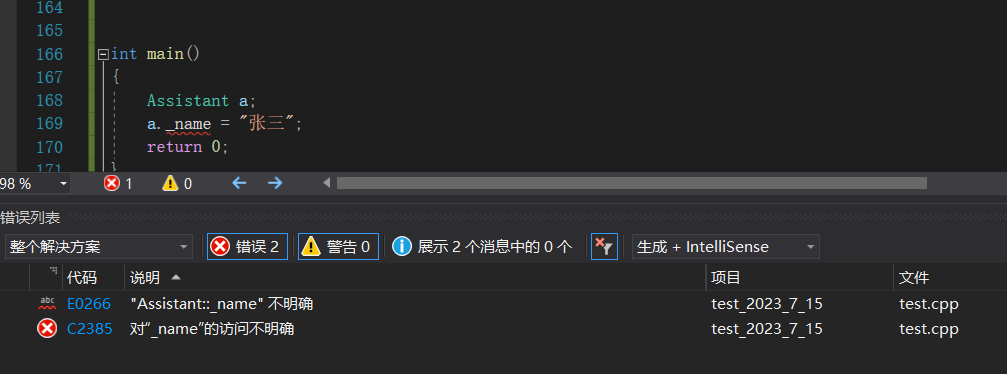int main()
{
Assistant a;
a.Student::_name = "张三";
return 0;
}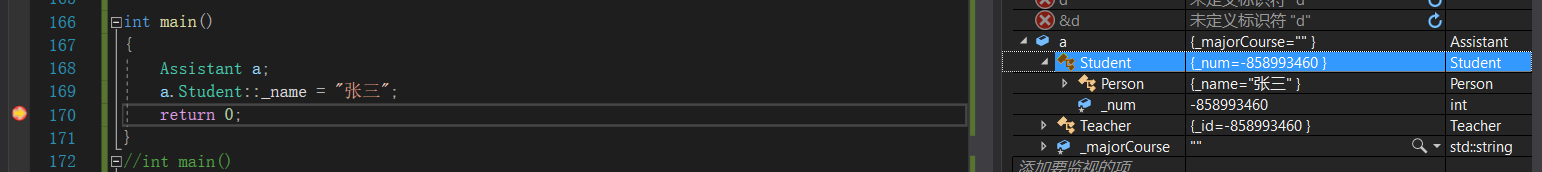## 7.虚拟继承（#####）

class A {
public:
int _a;
};

class B :public A
{
public:
int _b;
};

class C :public A
{
public:
int _c;
};
class D :public B ,public C
{
public:
int _d;
};

int main()
{
D d;
d.B::_a = 1;
d.B::_b = 2;
d.C::_a = 3;
d.C::_c = 4;
d._d = 5;

return 0;
}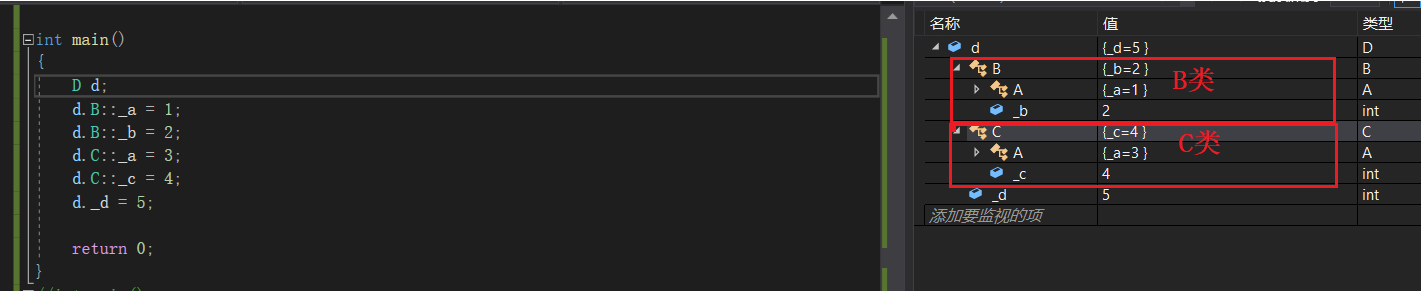class A {
public:
int _a;
};

class B : virtual public A
{
public:
int _b;
};

class C : virtual  public A
{
public:
int _c;
};
class D : virtual public B ,virtual public C
{
public:
int _d;
};

int main()
{
D d;
d.B::_a = 1;
d.B::_b = 2;
d.C::_a = 3;
d.C::_c = 4;
d._d = 5;

return 0;
}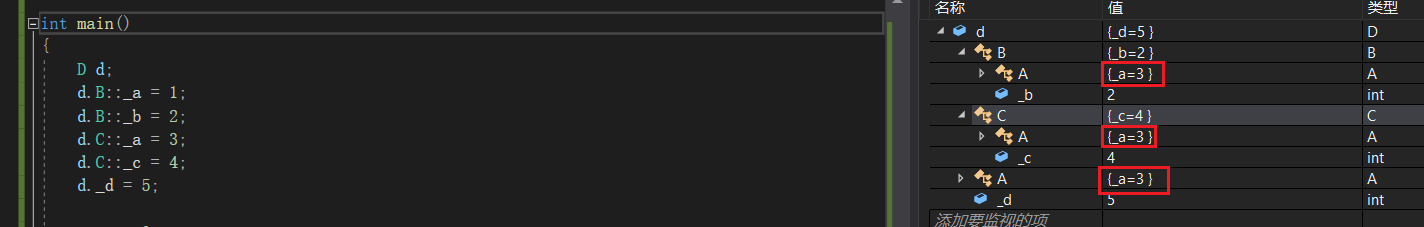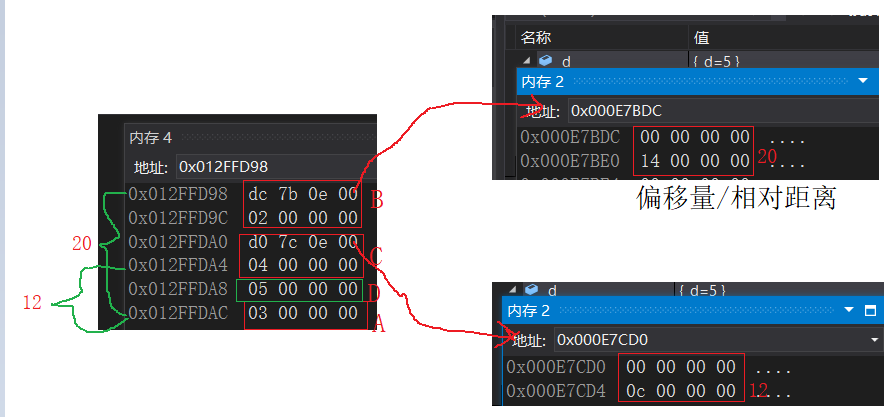## 总结

1. 很多人说C++语法复杂，其实多继承就是一个体现。有了多继承，就存在菱形继承，有了菱形继承就有菱形虚拟继承，底层实现就很复杂。所以一般不建议设计出多继承，一定不要设计出菱形继承。否则在复杂度及性能上都有问题。
2. 多继承可以认为是C++的缺陷之一，很多后来的OO（面向对象）语言都没有多继承，如Java。

public继承是一种is-a的关系。也就是说每个派生类对象都是一个基类对象。

is-a 的意思就是 子类（学生）就是一个父类（人） 白箱复用(white-box reuse)

has-a 的意思是 我在我的域中使用你 ，你是我的一部分，比如我使用我的鼻子，在STL库中，每个容器中的iterator迭代器 就是属于这样的关系，黑箱复

### 面试题

class A{
public:
A(char *s) { cout << s << endl; }
~A(){}
};

class B :virtual public A
{
public:
B(char *s1, char*s2) :A(s1) { cout << s2 << endl; }
};

class C :virtual public A
{
public:
C(char *s1, char*s2) :A(s1) { cout << s2 << endl; }
};

class D : public B, public C
{
public:
D(char *s1, char *s2, char *s3, char *s4) :B(s1, s2), C(s1, s3), A(s1)
{
cout << s4 << endl;
}
};

int main() {
D *p = new D("class A", "class B", "class C", "class D");
delete p;
return 0;
}


####################################################

## 结语|
2天前
|
C++
[C++/PTA] 狗的继承
[C++/PTA] 狗的继承
6 0
|
5天前
|

C++继承
C++继承
25 0
|
5天前
|

C++中的继承机制
C++中的继承机制
25 0
|
22天前
|
Java 编译器 C++
【C++从0到王者】第二十二站：一文讲透多继承与菱形继承（下）
【C++从0到王者】第二十二站：一文讲透多继承与菱形继承（上）
17 1
|
22天前
|

【C++从0到王者】第二十二站：一文讲透多继承与菱形继承（上）
【C++从0到王者】第二十二站：一文讲透多继承与菱形继承
9 1
|
22天前
|

【C++从0到王者】第二十一站：继承
【C++从0到王者】第二十一站：继承
17 1
|
24天前
|

C++继承
C++继承
6 0
|
26天前
|

C++ 继承下的构造函数和析构函数执行顺序
C++ 继承下的构造函数和析构函数执行顺序
11 0
|
26天前
|

C++ 怎么解决菱形继承
C++ 怎么解决菱形继承
14 0
|
1月前
|
C++
47 C++ - 继承中的静态成员特性
47 C++ - 继承中的静态成员特性
8 0